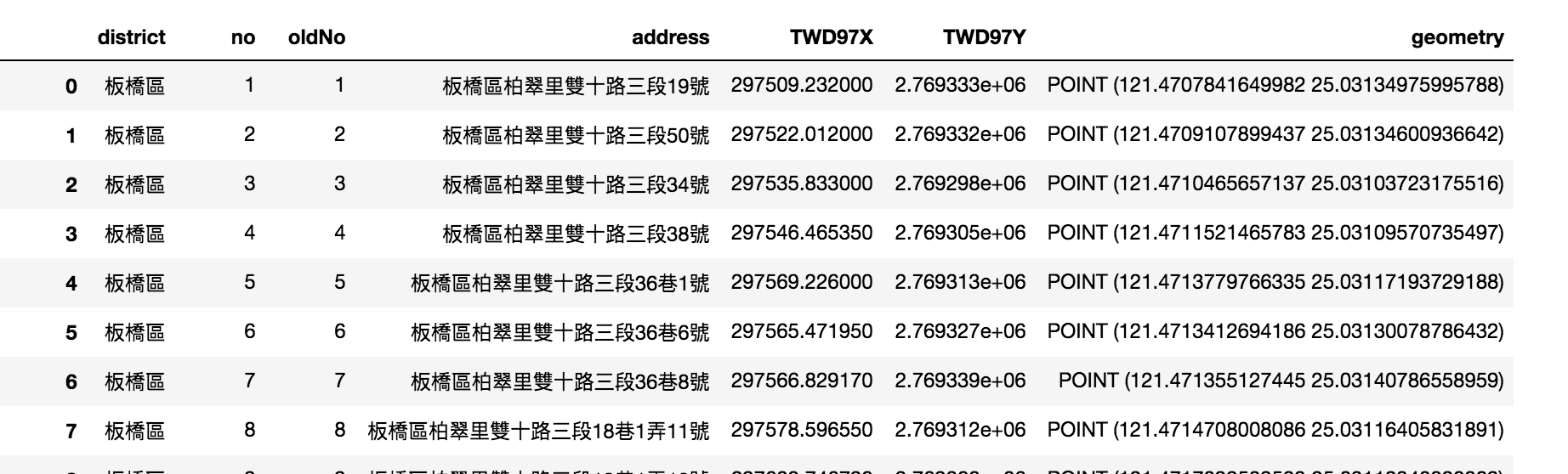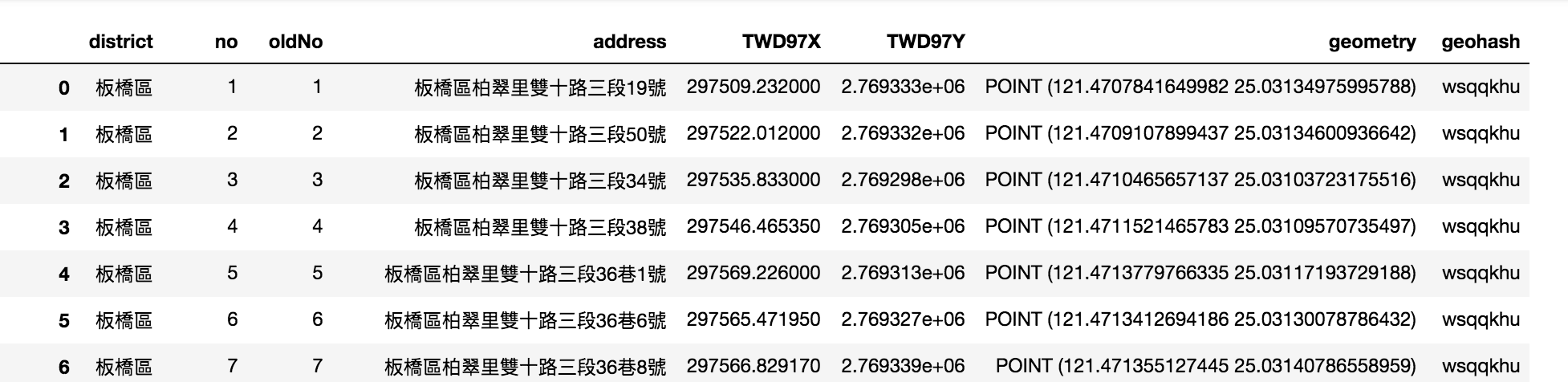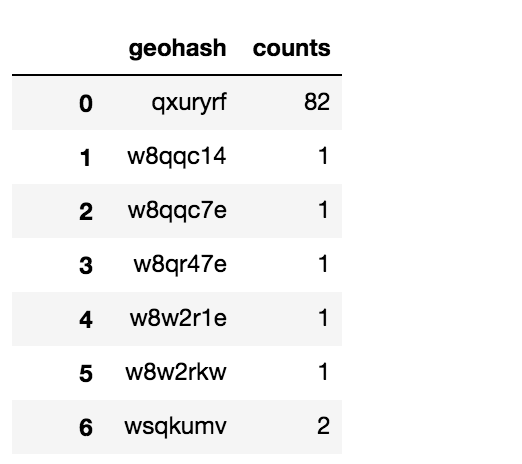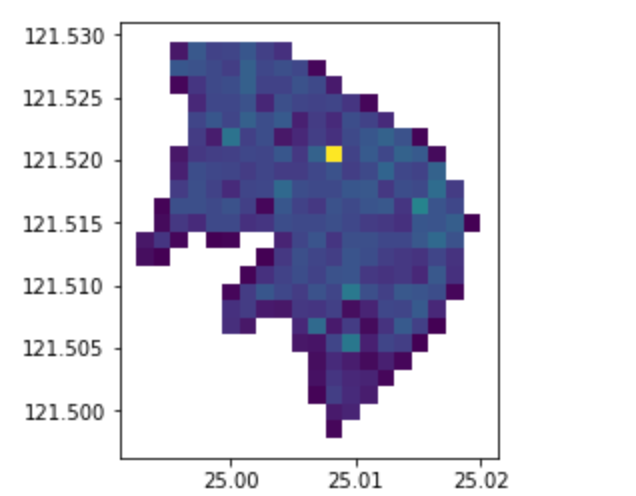#2019 iT 邦幫忙鐵人賽DAY 6
1
AI & Data

## Geohash

`geohash`故名意思是一種編碼，包含了多層級的架構，將空間劃分為網格(分為1-12層)，Geohash的產生是根據Z-order curve的方式產生的，這樣的特性讓查詢上可以透過hash編碼的前綴來加快空間搜尋速度。

`geohash`被很常用在GIS資料的indexing上，他有點類似R-tree或Quadtree，(取自GitHub - stephenlb/geohash-chat-by-proximity: Geo Hashing Chat by Proximity to connect two or more users to a group chat.)

geohash的每個層級的網格的解析度`大致`如下：
1 5,009.4km x 4,992.6km
2 1,252.3km x 624.1km
3 156.5km x 156km
4 39.1km x 19.5km
5 4.9km x 4.9km
6 1.2km x 609.4m
7 152.9m x 152.4m
8 38.2m x 19m
9 4.8m x 4.8m
10 1.2m x 59.5cm
11 14.9cm x 14.9cm
12 3.7cm x 1.9cm

Geohash encoding/decoding

GeohashExplorer

## 將點資料映射到geohash

### 轉為經緯度

`路燈`資料為例，先轉為經緯度資料

``````import geopandas as gpd
light=light[light.is_valid]
light=light[light['district']=='永和區']
light.crs = {'init' :'epsg:3826'}
light=light.to_crs(epsg=4326)
light
``````### geohash編碼

``````import geohash
light['geohash']=[geohash.encode(row['geometry'].y,row['geometry'].x, precision=7) for idx,row in light.iterrows()]
light

``````### 聚合

``````group=light.groupby('geohash')
group=group.size().reset_index(name='counts')
````````````from shapely.geometry import Polygon
geohashs=[]
for idx,row in group.iterrows():
decoded=geohash.bbox(row['geohash'])
geohashs.append(Polygon([(decoded['s'], decoded['w']), (decoded['s'],decoded['e']), (decoded['n'], decoded['e']), (decoded['n'],decoded['w'])]))
``````

``````g = gpd.GeoSeries(geohashs)
g_aggr = gpd.GeoDataFrame(group)
g_aggr['geometry']=g
g_aggr.plot('counts')
``````